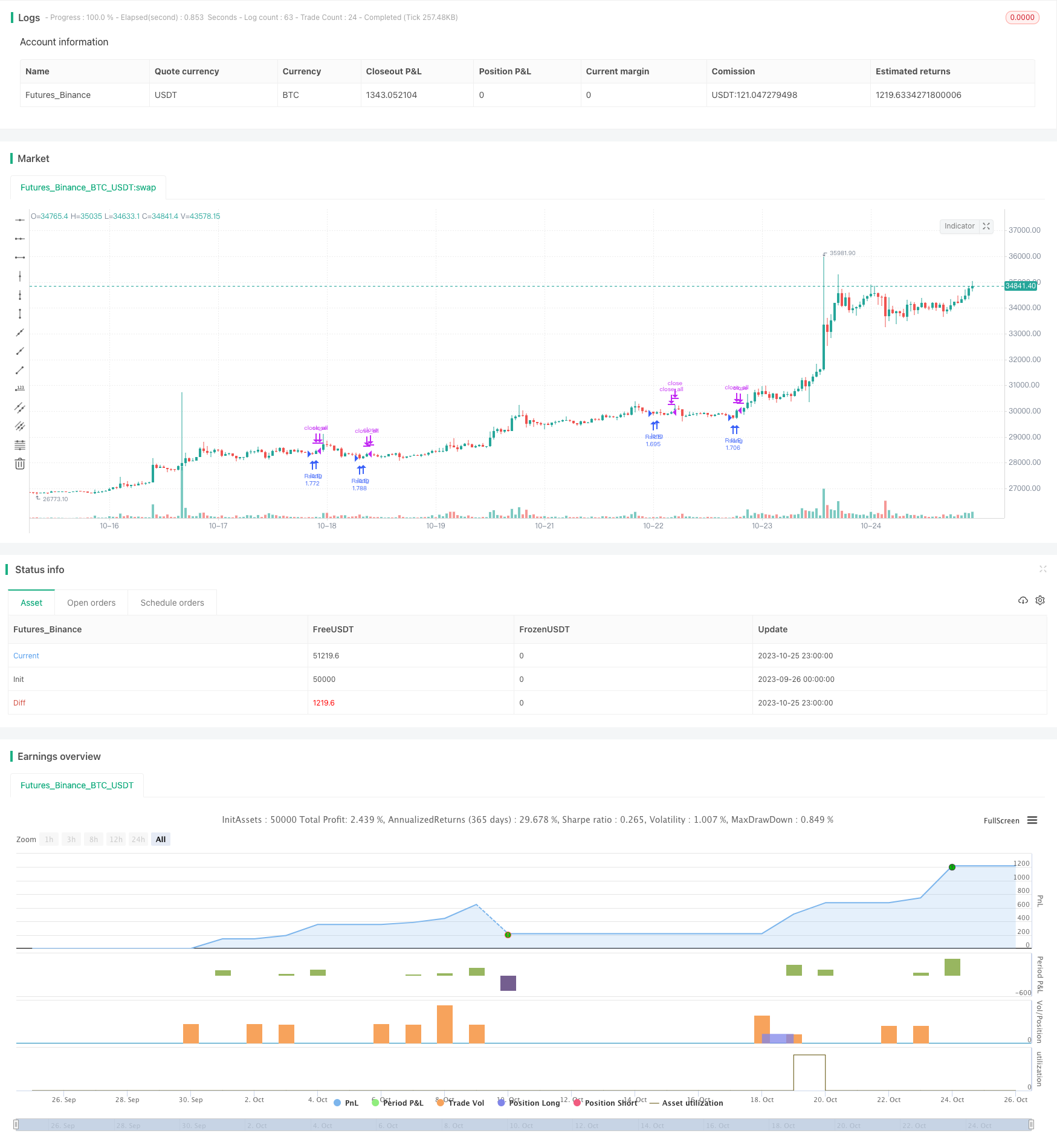# Oscillation Breakthrough Strategy

Author: ChaoZhang, Date: 2023-10-27 16:32:19
Tags:## Overview

This strategy is based on the classic idea of Larry Connors, using double moving average system to capture the medium-term oscillation of the market and take profit when it is overbought or oversold.

## Strategy Logic

1. Use 2-period RSI to determine if the price is in oversold region.

2. Use long period moving average (200 periods) to determine the major trend direction. Only consider opening position when price is above the long MA.

3. When price is above long MA and RSI is below oversold line, open long position at market price.

4. When price breaks through short period MA (5 periods) upwards, close long position at market price to take profit.

In addition, the strategy provides the following configurable options:

• RSI parameters: period length, overbought/oversold levels.

• MA parameters: long and short period.

• RSI MA filter: add RSI MA to avoid RSI fluctuation.

• Stop loss: configurable to add stop loss or not.

1. The double MA system can effectively track medium-long term trends.

2. RSI avoids missing the best entry timing during violent fluctuation.

3. Flexible configuration suitable for parameter optimization.

4. Breakthrough strategy, not likely to miss signals.

## Risk Analysis

1. Double MA strategy is sensitive to parameters, requiring optimization to achieve best performance.

2. No stop loss brings risk of expanding losses. Cautious position sizing is needed.

3. False breakout risks losses in oscillating market. Consider optimizing MA periods or adding other filters.

4. Backtest overfitting risk. Requires validation across markets and time periods.

## Optimization Directions

1. Test and optimize combinations of RSI and MA parameters to find optimum.

2. Test additional entry filters like volume spike to reduce false signals.

3. Add trailing stop loss to control single trade loss. Assess impact on overall profitability.

4. Evaluate impact of different holding periods to find optimal.

5. Test robustness in longer timeframes like daily.

## Summary

This strategy combines double MA trend tracking and RSI overbought/oversold to form a typical breakout system. With parameter optimization, strict risk management and robustness validation, it can become a powerful quantitative trading tool. But traders should beware of backtest overfitting and keep improving the strategy to adapt to changing market conditions.

```/*backtest
start: 2023-09-26 00:00:00
end: 2023-10-26 00:00:00
period: 1h
basePeriod: 15m
exchanges: [{"eid":"Futures_Binance","currency":"BTC_USDT"}]
*/

//@version=3
strategy("RSI Strategy", overlay=true, default_qty_type = strategy.percent_of_equity, default_qty_value = 100)

//Starter Parameters

length = input(title="RSI Lenght", defval=2)
overBoughtRSI = input(title="OverBought Level for RSI",  defval=10)
shortLength = input(title="Short MA Length",  defval=5)
longLength = input(title="Long MA Length",  defval=200)

RuleMRSI=input(title="RSI Moving Average Filter", defval= true)
lengthmrsi=input(title="RSI Moving Average Length",  defval=4)
overBoughtMRSI=input(title="OverBought Level for the Moving Average of the RSI",  defval=30)

Rulestop=input(title="Apply Stop Loss", defval=false)
stop_percentual=input(title="% Stop Loss",  defval=10)

//RSI

vrsi = rsi(close, length)

//Moving Averages

longma = sma(close,longLength)
shortma = sma(close,shortLength)
mrsi=sma(vrsi,lengthmrsi)

//Stop Loss

stop_level = strategy.position_avg_price*((100-stop_percentual)/100)

//Backtest Period
testStartYear = input(2009, "Backtest Start Year")
testStartMonth = input(1, "Backtest Start Month")
testStartDay = input(2, "Backtest Start Day")
testPeriodStart = timestamp(testStartYear,testStartMonth,testStartDay,0,0)

testStopYear = input(2020, "Backtest Stop Year")
testStopMonth = input(12, "Backtest Stop Month")
testStopDay = input(30, "Backtest Stop Day")
testPeriodStop = timestamp(testStopYear,testStopMonth,testStopDay,0,0)

testPeriod() => true

//Strategy

if testPeriod() and (not na(vrsi))
if  (RuleMRSI==false) and (Rulestop==false)
if (vrsi<overBoughtRSI) and (close>longma)
strategy.entry("RsiLE", strategy.long , comment="Open")
if (close>shortma)
strategy.close_all()

if (RuleMRSI==true) and (Rulestop==false)
if (vrsi<overBoughtRSI) and (close>longma) and (mrsi<overBoughtMRSI)
strategy.entry("RsiLE", strategy.long , comment="Open")
if (close>shortma)
strategy.close_all()

if (RuleMRSI==false) and (Rulestop==true)
if (vrsi<overBoughtRSI) and (close>longma)
strategy.entry("RsiLE", strategy.long , comment="Open")
strategy.exit("RsiLE", stop = stop_level)
if (close>shortma)
strategy.close_all()

if (RuleMRSI==true) and (Rulestop==true)
if (vrsi<overBoughtRSI) and (close>longma) and (mrsi<overBoughtMRSI)
strategy.entry("RsiLE", strategy.long , comment="Open")
strategy.exit("RsiLE", stop = stop_level)
if (close>shortma)
strategy.close_all()
```

More